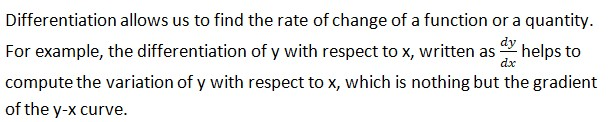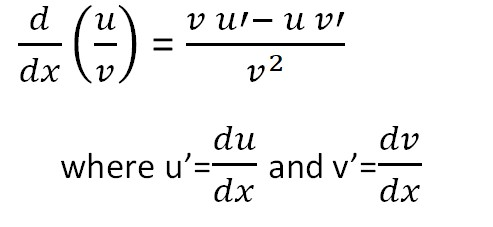Top

# GCSE Calculus

### INTRODUCTION

Calculus involves topics like limits, differentiation and integration that studies about the continuous changes between values that are related by a function. It helps in creating mathematical models to arrive at an optimal solution.

Let us study about differentiation and its specific rules

Differentiation:

Differentiation allows us to find the rate of change of a function or a quantity. For example, the differentiation of y with respect to x,written as helps to compute the variation of y with respect to x, which is nothing but the gradient of the y-x curve.

Rules of differentiation:

Calculas:2.Sum and difference rule:3.Product rule:4.Calculas 4:4.Chain rule: Consider the function F(x) to be the composite function defined by F(x) = f(g(x)) thenIntegration:

It is the reverse process of differentiation in which a function can be found out from its derivative.It is denoted by the symbol “ ∫ “. Integration can be used to find areas, volumes, central points and many useful things.

Rules of integration:

1.Multiplication by constant:

∫c f(x) dx = c∫ f(x) dx

2.Sum and difference rule:

∫(f(x) + g(x)) dx = ∫f(x) dx + ∫g(x) dx

∫(f(x) - g(x)) dx = ∫f(x) dx - ∫g(x) dx

3.Integration by parts:

∫ udv = uv - ∫ vdu

Choose u in this order : ILATE

Inverse Logs Algebraic Trigonometric Exponential

4.Substitution rule:

If the integral is of the form ∫ f(g(x) g’(x) dx we use this method whose value is given by

∫ f(g(x) g’(x) dx = F(g(x)) + c

Where F(x) = ∫f(x) dx